Latest Teaching jobs   »   UGC NET Previous Year Question JAN...

# UGC NET Previous Year Question JAN 2017 For Logical & Mathematical Reasoning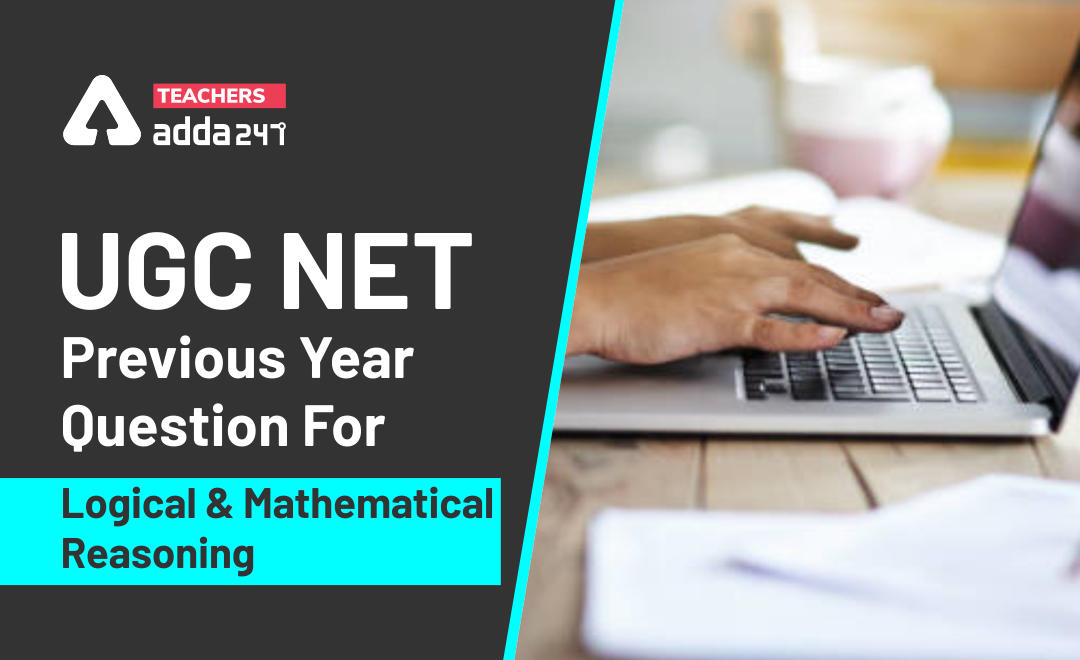Logical & Mathematical Reasoning are  important section in UGC NET Exam 2020.  UGC NET is an entrance exam for the selection of deserving candidates for the posts of Assistant Professor and Junior Research Fellowship (JRF).

Get free study Material for UGC NET 2020

## Highlights :

Exam Name UGC NET
Subject Reasoning (including Maths), Logical Reasoning, Data Interpretation
Total Questions 5 (for each)
Total Marks 10 (for each)
Marking For right answer – 2 mark
Negative Marking No
Question Pattern Objective type
Language Bilingual
Mathematical Reasoning and Aptitude
• Types of reasoning.
• Number series, Letter series, Codes and Relationships.
• Mathematical Aptitude (Fraction, Time & Distance, Ratio, Proportion and Percentage, Profit and Loss, Interest and Discounting, Averages etc.).
##### Logical Reasoning
• Understanding the structure of arguments: argument forms, structure of categorical propositions, Mood and Figure, Formal and Informal fallacies, Uses of language, Connotations and denotations of terms, Classical square of opposition.
• Evaluating and distinguishing deductive and inductive reasoning.
• Venn diagram: Simple and multiple use for establishing validity of arguments.
• Indian Logic: Means of knowledge.
##### Data Interpretation
• Sources, acquisition and classification of Data.
• Quantitative and Qualitative Data.
• Graphical representation (Bar-chart, Histograms, Pie-chart, Table-chart and Line-chart) and mapping of Data.
• Data Interpretation.
• Data and Governance.

Here we are going to provide you quizzes related to UGC NET syllabus which contains mainly flowing topics i.e. Reasoning (including Maths), Logical Reasoning, Data Interpretation

### Today Quiz : UGC NET Previous Yr Quiz Jan 2017

Q1. The next term in the following series YEB, WFD, UHG, SKI, ? will be

(a) TLO

(b) QOL

(c) QLO

(d) GQP

Q2. If A is coded as C, M as I, N as P, S as 0, I as A, P as N, E as M, 0 as E and C as S, then the code of COMPANIES will be

(a) SPEINMOAC

(b) NCPSEIOMA

(c) SMOPIEACN

(d) SEINCPAMO

Q3. Ali buys a glass, a pencil box and a cup and pays Rs. 21 to the shopkeeper. Rakesh buys a cup, two pencil boxes and a glass and pays Rs. 28 to the shopkeeper. Preeti buys two glasses, a cup and two pencil boxes and pays Rs. 35 to the shopkeeper. The cost of 10 cups will be

(a) Rs.40

(b) Rs.60

(c) Rs.80

(d) Rs.70

Q4. Out of four cities given below three are alike in some manner while the fourth one is different. Identify the odd one

(a) Lucknow

(b) Rishikesh

(d) Patna

Q5. Given below are some characteristics of reasoning. Select the code that states a characteristic which is not of deductive reasoning:

(a) The conclusion must be based on observation and experiment.

(b) The conclusion should be supported by the premise/premises.

(c) The conclusion must follow from the premise/premises necessarily.

(d) The argument may be valid or invalid.

Q6. The missing term in the series 1, 4, 27, 16, ?, 36, 343, … is

(a) 30

(b) 49

(c) 125

(d) 81

Q7. Among the following propositions two are related in such a way that they cannot both be true but can both be false. Select the code that states those two propositions.

Propositions:

(i) Every student is attentive.

(ii) Some students are attentive.

(iii) Students are never attentive.

(iv) Some students are not attentive.

Codes:

(a) (i) and (ii)

(b) (i) and (iii)

(c) (ii) and (iii)

(d) (iii) and (iv)

Q8. Given below are two premises ((a) and (b)). From those two premises four conclusions (i), (ii), (iii) & (iv) are drawn. Select the code that states the conclusions validly drawn from the premises (taking singly or jointly.)

Premises:

(I) Untouchability is a curse.

(II) All hot pans are untouchable.

Conclusions:

(i) All hot pans are curse.

(ii) Some untouchable things are hot pans.

(iii) All curses are untouchability.

(iv) Some curses are untouchability.

Codes:

(a) (i) and (ii)

(b) (ii) and (iii)

(c) (iii) and (iv)

(d) (ii) and (iv)

Q9. If the statement ‘None but the brave wins the race’ is false which of the following statements can be claimed to be true?

Select the correct code:

(a) All brave persons win the race.

(b) Some persons who win the race are not brave.

(c) Some persons who win the race are brave.

(d) No person who wins the race is brave.

The table below embodies data on the sales revenue (Rs. in lakh) generated by a publishing house during the years 2012-15 while selling books, magazines and journals as three categories of items. Answer questions 10 – 12 based on the data contained in the table.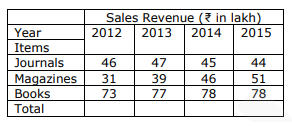Q10. The number of years in which there was an increase in revenue from at least two categories of items, is

(a) 0

(b) 1

(c) 2

(d) 3

Q11. If the year 2016 were to show the same growth in terms of total sales revenue as the year 2015 over the year 2014, then the revenue in the year 2016 must be approximately:

(a) Rs. 194 lakh

(b) Rs. 187 lakh

(c) Rs. 172 lakh

(d) Rs. 177 lakh

Q12. In 2015, approximately what percent of total revenue came from books?

(a) 45%

(b) 55%

(c) 35%

(d) 25%

A University professor maintains data on MCA students tabulated by performance and gender of the students. The data is kept on a computer hard disk, but accidently sonic of it is lost because of a computer virus. Only the following could be recovered: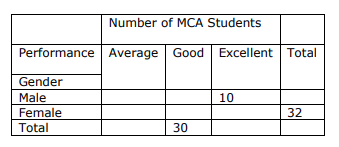Panic buttons were pressed but to no avail. An expert committee was formed, which decided that the following facts were self-evident:

(I) Half the students were either excellent or good.

(II) 40% of the students were females.

(III) One-third of the male students were average.

Answer questions 13 -15 based on the data given above:

Q13. What proportion of female students are good?

(a) 0

(b) 0.25

(c) 0.50

(d) 0.75

Q14. Approximately, what proportion of good students are male?

(a) 0

(b) 0.73

(c) 0.43

(d) 0.27

Q15. How many female students are excellent?

(a) 0

(b) 8

(c) 16

(d) 32

How to Crack UGC NET While Working?

Solutions
S1. Ans.(b)
Sol. YEB, WFD, UHG, SKI, _____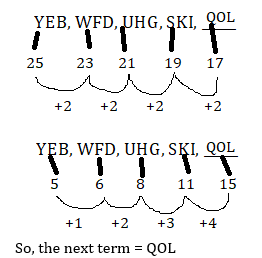S2. Ans.(d)
Sol. A is coded as C, M as I, N as P, S as 0, I as A, P as N, E as M, 0 as E and C as S,
So, code of COMPANIES  = SEINCPAMO
S3. Ans.(d)
Sol. Acc, to question,
Cost of 1 glass, 1 pencil box and 1 cup = Rs. 21
Cost of 1 glass, 2 pencil box and 1 cup = Rs. 28
Cost of 2 glass, 2 pencil box and 1 cup = Rs. 35
So, the cost of 1 cup = Rs. 7, cost of 1 glass = Rs. 7 and 1 cup = Rs. 7
the cost of 10 cups = 7 × 10 = Rs. 70
S4. Ans.(a)
Sol. Lucknow is the odd one out as all the rest of three cities are situated on the bank of holy river “Ganga” and lucknow is located on the bank of River gomti.
S5. Ans.(a)
Sol. (a) The conclusion must be based on observation and experiment.
S6. Ans.(c)
Sol.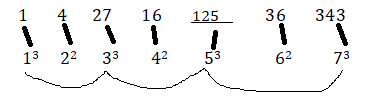S7. Ans.(b)
Sol. (i) Every student is attentive.
(iii) Students are never attentive.
S8. Ans.(d)
Sol. (ii) Some untouchable things are hot pans.
(iv) Some curses are untouchability.
S9. Ans.(b)
Sol. (b) Some persons who win the race are not brave.
S10. Ans.(c)
Sol. During 2012-2013 revenue increase in all catagories
During 2013-2014 revenue increase in magazines and books category
S11. Ans.(d)
Sol. Let the total sales in 2016 = A
IF % wise the growth is same for 2014-15 and 2015-16 then, the ratio should also be same.
Sales of (2014 ÷ 2015) = sales of (2015 ÷ 2016)
(169 ÷ 173) = (173 ÷ A)
A = (173 × 173) ÷ 169
A=13.3 x 13.3
A=~177 lakh
S12. Ans.(a)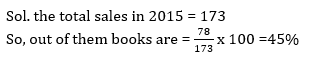S13. Ans.(b)S14. Ans.(b)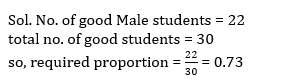S15. Ans.(a)
Sol. Acc. to question, half the students are either good or excellent
total no. of good students = 30
no. of male excellent students = 10
so, no. of female excellent students = 0

Get free study Material for UGC NET 2020

For More Details :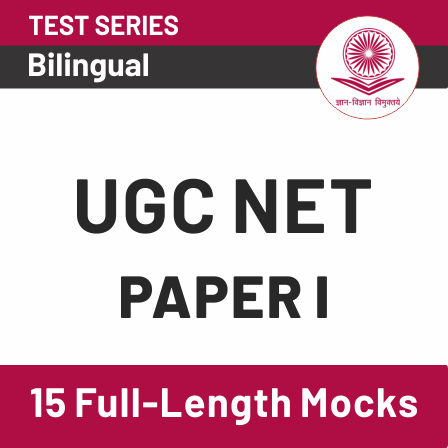Sharing is caring!

Thank You, Your details have been submitted we will get back to you.